# Even graphs

Relevant articles: , , , , , , , , , , .

[hide]

# Even graphs

A graph is even if each vertex has even degree.

A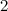$2$-regular graph is a union of vertex disjoint cycles.

We denote by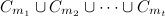$C_{m_1}\cup C_{m_2}\cup\cdots\cup C_{m_t}$ the$2$-regular graph consisting of$t$ vertex disjoint cycles of lengths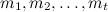$m_1,m_2,\ldots,m_t$.

An even graph which is connected is called a closed trail, and a closed trail with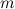$m$ edges is a called a closed$m$-trail.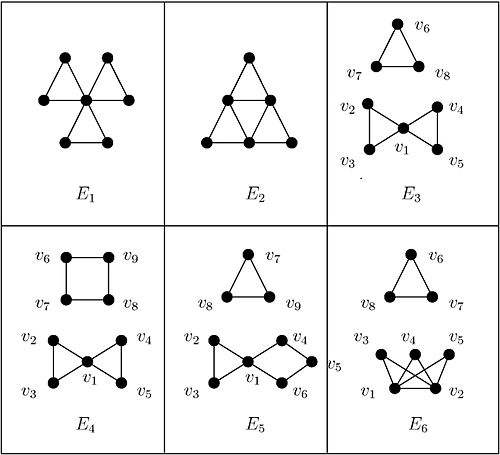## Spectrum Results

Table 1 summarises the known results on the spectrum problem for even graphs with up to ten edges. An explanation of the sources of these results is given in .

Table 1$m$ Spectrum for each even graph with$m$ edges Exceptions (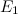$E_1$ and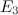$E_3$ as in Figure 1)$3$$n \equiv 1 {\rm \ or \ } 3 \,({\rm mod \ }6)$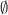$\emptyset$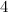$4$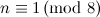$n \equiv 1 \,({\rm mod \ }8)$$\emptyset$$5$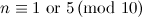$n \equiv 1 {\rm \ or \ } 5 \,({\rm mod \ }10)$$\emptyset$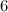$6$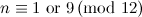$n \equiv 1 {\rm \ or \ } 9 \,({\rm mod \ }12)$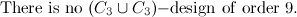${\rm There \ is \ no \ }(C_3 \cup C_3){\rm-design \ of \ order \ }9.$$7$$n \equiv 1 {\rm \ or \ } 7 \,({\rm mod \ }14)$$\emptyset$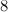$8$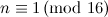$n \equiv 1 \,({\rm mod \ }16)$$\emptyset$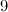$9$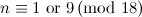$n \equiv 1 {\rm \ or \ } 9 \,({\rm mod \ }18)$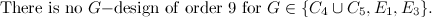${\rm There \ is \ no \ }G{\rm-design \ of \ order \ }9{\rm \ for \ }G\in\{C_4 \cup C_5, E_1, E_3\}.$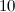$10$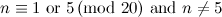$n \equiv 1 {\rm \ or \ } 5 \,({\rm mod \ }20){\rm \ and \ }n\neq 5$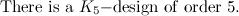${\rm There \ is \ a \ }K_5{\rm-design \ of \ order \ }5.$

Theorem 1 

Let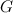$G$ be a$2$-regular bipartite graph. There exists a$G$-design of order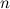$n$ for all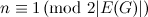$n \equiv 1 \,({\rm mod \ }2|E(G)|)$.

Theorem 2 

Let$G$ be a$2$-regular graph with at most three components. There exists a$G$-design of order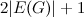$2|E(G)| + 1$.

Theorem 3

Let$G$ be the union of$t$ vertex disjoint$3$-cycles.

• There exists a$G$-design of order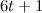$6t+1$ .
• If$n \equiv 1 {\rm \ or \ } 3 \,({\rm mod \ }6)$ and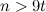$n > 9t$ there exists a$G$-design of order$n$ .

Theorem 4 

Let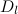$D_l$ be the union of$l$ edge-disjoint$3$-cycles each sharing a common vertex. If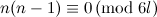$n(n-1) \equiv 0 \,({\rm mod \ }6l)$ and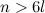$n>6l$ then there exists a$D_l$-design of order$n$.

## Notes

• It is well-known (though at present there is no published proof, see ) that there is no$(C_3\cup C_3\cup C_5)$-design of order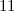$11$.
• Results on the famous Oberwolfach problem provide further$G$-designs of orders$n$ for many instances where$G$ is a$2$-regular graph with$n$ vertices, see the section on unions of graphs.
• When$3\leq m\leq 5$ the only closed$m$-trails are cycles (see the section on cycles).
• When$m=6$ we have only the$6$-cycle and the graph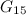$G_{15}$ (see Figure 1 in the section on Graphs with five vertices).
• A Dutch$l$-windmill, denoted$D_l$, is the graph containing a vertex,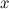$x$ say, such that$D_l$ is the union of$l$ edge-disjoint 3-cycles, all of which contain the vertex$x$.
• There are four even graphs with$10$ or fewer vertices which are neither$2$-regular nor closed trails, namely the graphs$E_3$,$E_4$,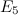$E_5$ and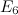$E_6$ shown in Figure 1.

## References

1. 1.0 1.1 Adams, P., Bryant, D., and Buchanan, M. A survey on the existence of G-designs, J. Combin. Des. 16, 373–410 (2008).
2. Adams, P., Bryant, D., and Gavlas, H. Decompositions of the complete graph into small 2-regular graphs, J. Combin. Math. Combin. Comput. 43, 135–146 (2002).
3. Adams, P., Bryant, D. E., and Khodkar, A. The spectrum problem for closed m-trails, m\leq 10, J. Combin. Math. Combin. Comput. 34, 223–240 (2000).
4. 4.0 4.1 Aguado, A., El-Zanati, S. I., Hake, H., Stob, J., and Yayla, H. On ρ-labeling the union of three cycles, Australas. J. Combin. 37, 155–170 (2007).
5. 5.0 5.1 Alspach, B. The Oberwolfach problem, CRC Press Series on Discrete Mathematics and its Applications, The CRC handbook of combinatorial designs, ed. 1, CRC Press, 394–395 (1996).
6. Bermond, J. -C., Huang, C., Rosa, A., and Sotteau, D. Decomposition of complete graphs into isomorphic subgraphs with five vertices, Ars Combin. 10, 211–254 (1980).
7. 7.0 7.1 Blinco, A. and El-Zanati, S. I. A note on the cyclic decomposition of complete graphs into bipartite graphs, Bull. Inst. Combin. Appl. 40, 77–82 (2004).
8. 8.0 8.1 Dinitz, J. H. and Rodney, P. Disjoint difference families with block size 3, Util. Math. 52, 153–160 (1997).
9. 9.0 9.1 9.2 Horák, P. and Rosa, A. Decomposing Steiner triple systems into small configurations, Ars Combin. 26, 91–105 (1988).
10. Köhler, E. Über das Oberwolfacher Problem, Lehrbücher Monograph. Geb. Exakten Wissensch., Math. Reihe, Beiträge zur geometrischen Algebra (Proc. Sympos., Duisburg, 1976), Birkhäuser, Basel, 189–201 (1977).
11. Mullin, R. C., Poplove, A. L., and Zhu, L. Decomposition of Steiner triple systems into triangles, Proceedings of the first Carbondale combinatorics conference (Carbondale, Ill., 1986), 149–174 (1987).